SELF 62 S.B. Karavashkin and O.N. KaravashkinaThus, breaking the interrelation between the geometric axioms on one hand and of mathematical formalism of physics on the other – and the experience, relativists in their imagination got a full freedom to manipulate with physical symbols irrespectively of the physical meaning that was initially founded in them and bringing the physical and mathematical logic to a complete absurd: “ The general theory of relativity has brought even deeper changes that threw away the concept of inertial frame, at all. In the previous theories, space mathematically expressed by the inertial frame was considered as an independent element of physical reality. One could consider this element as something absolute, as it determined the behaviour of pointed masses or field which did not affect it themselves. However, in the general theory of relativity the inertial frame is substituted by the field of shifts, which is a component of the unified field being the only mean to describe the real world (?! – Authors). The spatial aspect of real things, thus, is fully expressed by the field dependent on the four coordinates – parameters; it is the property of this field. If we imagine that the field is removed, the ‘space’ also will not remain, as the space has no independent existence” [6, p. 787–788]. The inner meaning of relativistic substitution of space by the power field becomes obvious if we account the known feature of vectors: “Now let us mark the essential feature of methods to study ‘the field theory’. In this subject, as long ago as in 19th century, the scientists began to feel about the main ‘nucleus’ having a mathematical pattern. This ‘nucleus’ contains the following essential concepts: gradient, potential, flux, divergence, curl, circulation and some other. To know these concepts is in a great necessity in studying physics, mechanics and some other engineering disciplines. But the same concepts are important in learning the basic ideas of mathematical analysis of the functions of many variables and, in amount with some other geometric forms, they as if invest many abstract ideas of mathematical analysis with a body” [43, p. 5]. Thus, the difference between the vectorial description of a field on one hand and of space on another is that in the power field the vectors describe the body-to-body interaction, while in space the vectors describe only the mutual location of bodies, not their interaction. And this in no way can be thought identical, as well as we may not adequately substitute the description of field by the study of geometric trajectories of particular bodies; even less we may do so in non-inertial frames that distort the true accelerations of the studied bodies. Just so, to describe the power interaction, we have to use, together with the physical laws of body-to-body interaction, the coordinates that determine the location of interacting bodies and the inertial frame which gives a full pattern to the process of acceleration. Relativists have violated this rule, illegally joining these vectorial descriptions of space and power field: “In the general theory of relativity, we give much more physical meaning to the ratio of components of potential of gravity field gthan to the very components g. It is explained by the fact that the amount of world lines originating from some point of the universe along which the light signals can propagate from this point, or the light sphere, seems to be determined by the very space-time continuum; and the sphere is determined by the equation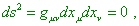which contains not only the ratio g. Generally, only ratios gare included to the equations of electromagnetic field in vacuum. While the value ds that can be determined only through gdoes not express any properties of space-time continuum, because to measure these values, we need a material object (clock). So the question inevitably arises: cannot we change the theory of relativity in supposition that not the value ds as such is invariant but only the relation ds2 = 0 ?” [44, p. 105]. As we see, Einstein makes far going general premises, proceeding from the outer likeness in the geometric shape of the force lines of central field in the particular problem of theory of potential and the structure of metric of space in the spherical coordinates, and neglecting the features of structure of vectors in these constructions. Neglecting, he automatically contradicts all laws of vector algebra, nothing to say of physical laws of the power field. Moreover, stating that “only ratios gare included to the equations of electromagnetic field in vacuum. While the value ds that can be determined only through g”, Einstein confirms that in his theory the power field is given not through the coordinates plus the values of vectors of this field’s strength at this point but exceptionally through the coordinates in the mythic curved space. In turn, as we pointed above, this brings relativists to the mystification of the force interaction: “The power field is, consequently, the divergence between the natural geometry of the coordinate system and abstract geometry that is arbitrarily attributed to it. The power field arises, thus, due to a definite arrangement of our mind. Should the assumed coordinate system was not interpreted wrongly comparing with what it really is, the power field would not exist. If we do not consider our rotating axes so as if they were non-rotating, there would arise no centrifugal force” [7, p. 71]. At the same time, as Einstein himself admitted, “according to the general theory of relativity … if the frame K'  rotates uniformly relative to the inertial frame K, the material points move relative to K' so that the acceleration depends not only on their location (the centrifugal force) but on the speed (the Coriolis force)” [45, p. 423]. As we can easily see, when a specific problem, relativists recall the concepts of forces and features of motion of a material body in non-inertial frames, and inertial frames come to light, passing to which, we can reveal the regularities of centrifugal force and Coriolis force, which corroborates again how artificial is the relativistic mystification. To see the inner meaning of such mystification of power interaction, let us proceed from the opposite and premise that the power interactions do not exist – it is the curvature of space what exists and substitutes the track of material particle. Furthermore, premise that we have the same curved surface on which the material body is located, as we se it in Fig. 5.2.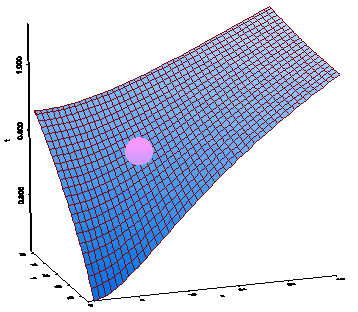Fig. 5.2. A material body on the curved surface
 As we can see, the surface is not only curved but considerably inclined towards the coordinate origin. Does it follow from this that the material body will slip down to the coordinate origin? No, of course. The body to move, something has to make it moving. Can the surface make it? No, also. But if this surface is, for example, in the gravity field of the Earth, then the body will slip sown. However, the pattern will basically change, as Fig. 5.3 shows.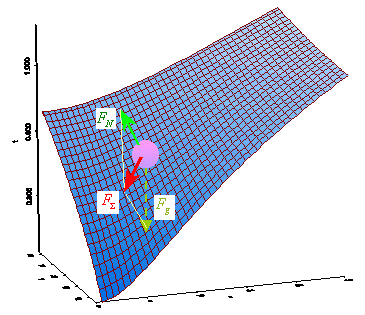Fig. 5.3. A material body on the curved surface in the outer gravity field
 As we can see from the construction, the body’s motion is determined even not by the only gravity force Fg  but by two forces: Fg  and the reaction of surface FN  without which the body would move with Fg , ignoring the curvature of surface. Thus, if we limit the description of body’s behaviour in the outer field only by the trajectory of this body, in absence of forces the trajectory will be absent, and the body will rest infinitely long time. Again, if we premise that the body had an initial speed, then, in order its trajectory to curve, this metric has to affect, and the force has to exist which would create the reaction of the surface of metric. But if relativists claim the forces to be imaginary, because of which the reaction of surface and the centrifugal force in Eddington’s works are mythic, the body’s trajectory has no grounds to curve. And when we modelled the surface as some material extensive body able to react to the external affection, we automatically premised that the body is able to interact with this surface. In space we may not premise such interaction of our Riemann metric with the material body. While interaction through the bodies is a multiply checked experimental fact. Thus, “both here and in any other area of Theoretical physics we should differ, on one hand, general ideas and hypotheses of physical kind, and on the other – a set of mathematical formulas and calculations with whose help these ideas and hypotheses can be expressed and worked out” [46, p. 19]. In this connection we have to differ the spatial vectors and force vectors. Not in vain Maxwell, when introduced his definition of vector, even introduced a special concept ‘the function of vector’. He defined as a vector the initial concept of spatial vector whose value is determined between two selected points of space, and as a function of vector – a directed value determined at the very point through the force affection onto a trial body: “in the quarternion calculus, the spatial location of a point is determined by the vector drawn to this point from some fixed point called the initial point, or the coordinate origin. If we had to study some physical entity whose value depends on the location of point, it is considered as the function of vector drawn from the coordinate origin. This function can be both scalar and vectorial. The density of a body, its temperature, its hydrostatic pressure, potential at the point – these all are examples of scalar functions. The resulting force at the point, the speed of liquid at the point, the rotation speed of an element of liquid, as well as the moment of a pair of forces that produce rotation, – these all are examples of vector functions” [10, p. 36]. Just due to it, even if we outwardly accept the field lines similar to some curved surface, this never will be able to describe really the force interaction. And Einstein admitted it when wrote in the beginning of his work “On the general theory of relativity”: “The postulate of relativity in the measure in which I required is true always when the principle of Hamilton is put as the basis; however factually it disables to determine the Hamiltonian function H of the gravity field. Factually the relationship … that limits the choice of H expresses nothing more than the fact that H has to be invariant relative to the linear transforms, and this requirement has nothing in common with the relativity of acceleration” [47, p. 425]. And this is well seen on the above example of a material body on the curved surface. If we change the direction of force or its value, the body on the same curved surface will move otherwise and with other acceleration. If, changing the parameters of force vectors, we change also the curvature of surface, we have to provide, the body to have the same location at the initial moment in the initial 3-D space into which the curved surface is nested. Extending the problem onto the set of bodies located at some region of space, we put the location of each body in an insoluble question when changing the metric of this curved surface which relativists associate with the external affection of space-time. Actually, on one hand, according to the relativistic opinion, “we may freely use the concepts of 4-D geometry and of imaginary rotations to find this relation, irrespectively of, has or not this representation whatever physical meaning” [7, p. 36]. It follows from such unscrupulousness of methods that “in the curved space-time, thus, there is no reference system which would appear everywhere an inertial or everywhere a rectilinear coordinate system. A freely falling frame is a coordinate system whose axes are geodetics, but only in a small vicinity of the world point. If we extend such reference system over the given region, it will cause the fact that the reference system already does not differ from any other curvilinear coordinate system; in the curved multiplicity any smooth enough (though curved) coordinate system is the same acceptable as all others” [48, p. 81]. Moreover, “the curved multiplicities have definite geometric properties having nothing in common with the choice of coordinate system” [ibidem]. And at the same time, “all these, as well as other properties of multiplicities can be described in any coordinate system. By understandable reason, the kind of this description is changed in passing from one coordinate system to another” [48, p. 82]. But the main, as relativists think, is the following. “The curved space-time in no way contradicts the understanding of freely falling reference frame. To say geometrically, a freely falling frame is a local coordinate system determined only in the immediate vicinity of the world line; the coordinate points of such system are almost direct lines perpendicular to each other; this is in a full agreement with the presence of space-time curvature at all world points. Gravity fields always are inhomogeneous; the field strength and acceleration caused by the field change in passing from point to point. Just by these reasons, the freely falling reference frame cannot be extended without limits” [48, p. 80–81]. Let us draw our attention here: Einstein says not of any accelerated frame but exceptionally of a frame freely falling in the outer gravity field; we will analyse the reason of it in the next section. So Einstein outwardly says of some generalisation but factually reduces the problem again to the particularity convenient for him, trying, just as he did it when formulated the basis of SRT, in order to add to this particular an appearance of a holistic law of nature. And reduced because in other particularities his equivalence of inertial and non-inertial frames is invalid even more than in case of freely falling frame. For example, as we showed in [13, p. 15], in the local relativistic non-inertial frame the body that is not on the axis connecting the origin of frame with the gravitating body will move nonlinearly, and relativists well understood it: “If we try to extend this frame to some distance, other freely falling trial bodies … will have some acceleration relative to this frame; this acceleration will be in proportion to the distance from the place where we built the initial freely falling frame” [48, p. 87]. And if we assume the inertial and non-inertial frames equivalent, this curvilinear motion will have no cause and will not coincide with the geodetic. Moreover, if we substitute the power field by the geometry of polar coordinates, as for example Schwarzschild made in the statement of his problem which he solved by Einsteinian methods: The field equations with the conditions for the determinant have a basic property that they remain their appearance in substituting the variables x1 , x2 , x3 , x4 by any other variables, if only the related Jacobian is equal to 1 … If we denote the time as t and the rectilinear coordinates as x, y, z, then, obviously, the most general linear element that satisfies the requirements 1 – 3 (stated for the Einsteinian metric substituting the power field – Authors) is the following: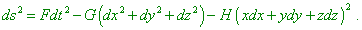Here F, G and H are the functions of the value r = sqrt ( x2 + y2 + z2 )… Passing to the spherical coordinates … yield for the same linear element the expression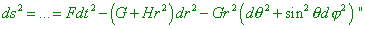(5.3)
 [49, p. 200–201], – in such metric the length of arc being the degree of polar decreases with the lessening of radius. With it, in the relativistic local frame the metric also would have to decrease in the same relation, in proportion as the frame approaches to the gravitating body, and with it the size of body had to diminish. And this change had to be real and independent of the size of the gravitating body, just as it had not to be dependent on the speed of this body, as such regularity – decreasing length of the arc – is typical for all polar coordinate systems, and in relativistic understanding, “in the gravity fields there do not exist the solid bodies with Euclidean properties; so the concept of a solid body of reference is not applicable in general theory of relativity, … So non-rigid bodies of reference are used, which can not only move arbitrarily as the whole but encounter the change of shape in their motion… This deformable body of reference which not without grounds we can call ‘a mollusc of reference’ is in essence equivalent to any 4-D Gaussian coordinate system” [50, p. 580]. Due to such identification, we would have a possibility to see the projective properties of material bodies whose size would not decrease for us with the height, and we would see the satellites distinctively, as if they are in the very near. We clearly don’t experience such things – consequently, it is basically incorrect to identify the power field with geometry of some reference frame, and when studying the field, we have to use the trivial approach which is used by classical physics, i.e. vectorially representing the spatial location of a trial body plus vectors of the power interaction at each point of the field. Then there appears the generality of solutions applicable to any initial conditions, location and type of motion of the studied body. And the advantage of polar coordinate system is not limited only by the fact that in these coordinates the expressions describing the interaction of bodies with the central field gain a simplest appearance convenient for operation. But geometry of polar coordinates does not, and is not able, to substitute the physical interaction, as well as the forces of interaction are determined in accordance with Maxwellian definition as the functions of spatial vectors.

Contents: / 53 / 54 / 55 / 56 / 57 / 58 / 59 / 60 / 61 / 62 / 63 / 64 / 65 / 66 / 67 / 68 / 69 / 70 / 71 / 72 / 73 / 74 / 75 / 76 / 77 / 78 / 79 / 80 / 81 / 82 / 83 /